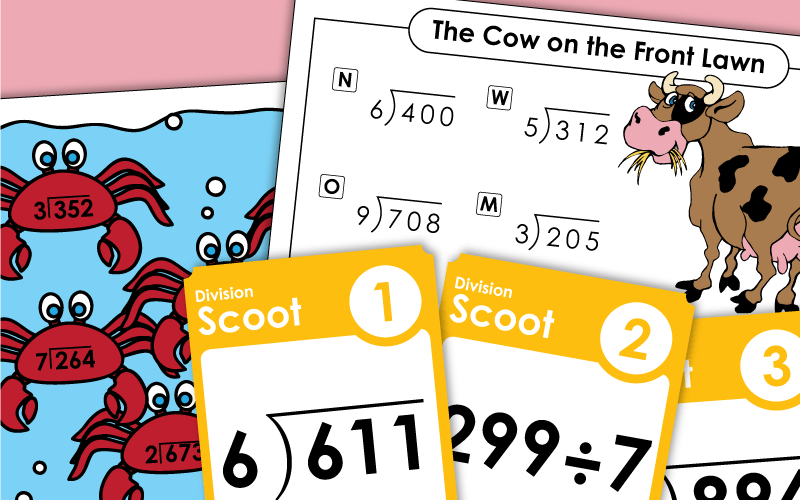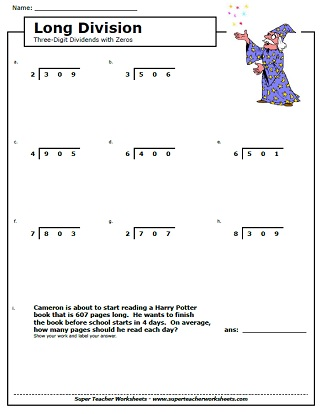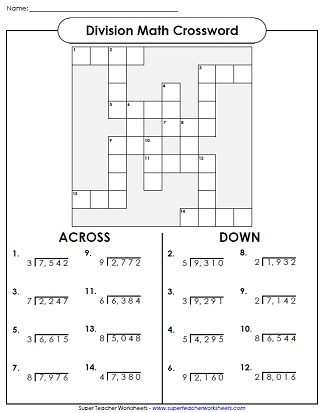# Long Division with 3-Digit Dividends

This page has long division printables. Problems in this series have 3-digit dividends and 1-digit divisors. Includes task cards, word problem worksheets, and drills, and puzzles.## 3-Digit Dividends

3-digit dividends, 2 or 3-digit quotient, all problems have remainders; Goldfish picture and word problem.
This worksheet includes various types of division questions, including word problems and a table to complete.
This variety worksheet features different types of division questions involving remainders.
Find the answers to the division problems and color the quotients on the bingo board.  Can you get a bingo?  (3-digit dividends and 2-digit quotients without remainders.)
This worksheet has a math riddle for students to solve. What do you call a sleeping bull? To decipher the answer, students will solve division problems with 3-dig dividends and 2-dig quotients. These problems do not have remainders.
What do you call a cow on your front lawn? The answer to the riddle can be solved by completing division problems. Each problem has a 3-digit dividend. All quotients have remainders.
This printable division activity features 30 division problems listed traditionally, horizontally, and as fractions with 2 and 3 digit dividends.
Divide the problem on each task card. Each problem has a 3-digit dividend and a 1-digit divisor. (example: 126 divided by 6) These cards can be used for classroom scavenger hunts, learning centers, document readers, or small group instruction.
This worksheet features division problems with 3-digit dividends with remainders. Sea animal pictures illustrate the worksheet.
Division problems with 3-digit dividends; some have remainders; Students need to re-write the problem in long division form.
Student rewrite each long division division problem and solve. Each problem has a 3-digit dividend.
Solve the long division problems (no remainders) and attach the puzzle pieces in the correct places.  Scene shows a girl ski jumping.
At the top of the worksheet, students are shown a series of shapes with numbers in them. They divide numbers in similar shapes.
Complete these long division word problems with 3-digit dividends.
Students will use the cypher key to decode the dividend and divisor in each problem. Then they'll solve. These problems have 3-digit dividends, 2-digit divisors, and no remainders.
THis crypto-code math worksheet has 3-digit dividends and 2-digit quotients. Each problem has a remainder.
Worksheet featured 3-digit dividends with 2-digit quotients; Some quotients have remainders.
Division problems with 3-digit dividends and 2-digit quotients.
Division problems with 3-digit dividends and 3-digit quotients.
In this whole-class game, students will move from place to place finding division cards. They solve the problem on the card and wait for the leader to yell, "Scoot!" Then they go to the next card.

## Long DivisionWorksheet Generator

Division Worksheet Generator

Create your own long division worksheets! You choose the number of digits in the dividend and the divisor.

## Quotients with Zero

Long division:  3-digit quotients with zeros; some remainders; Car picture and word problem.
3-digit dividends with zeros in the tens place, with remainders; Wizard picture and word problem.
Students rewrite each problem in long division form and solve. Each quotient has a zero in it.
More Long Division

Find printables for reviewing division with 2, 3, or 4-digit dividends, as well as 2-digit divisors. You'll also be able to access worksheets for dividing money and fractions.

## Sample Images ofLong Division WorksheetsMy Account
Site Information